## Figures index

#### C. I. Nwoye, P. C. Agu, B. C. Chukwudi, S. O. Nwakpa, I. A. Ijomah, N. E. Idenyi

Biomedical Science and Engineering. 2015, 3(1), 15-22 doi:10.12691/bse-3-1-4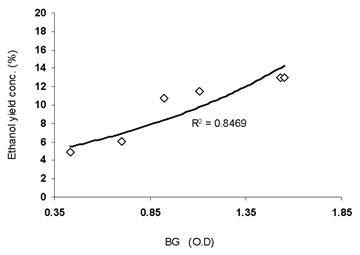• Figure 1. Coefficient of determination between ethanol yield and microbial growth as obtained from experiment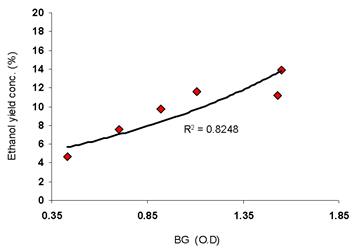• Figure 2. Coefficient of determination between ethanol yield and microbial growth as predicted by derived model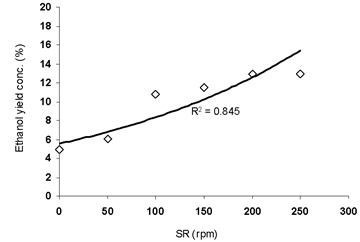• Figure 3. Coefficient of determination between ethanol yield and shaking rate as obtained from experiment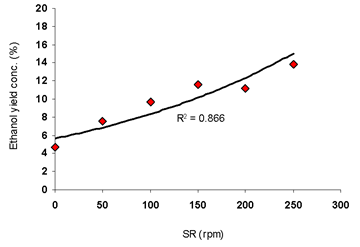• Figure 4. Coefficient of determination between ethanol yield and shaking rate as predicted by derived model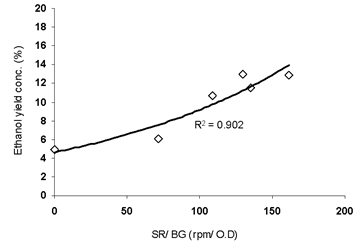• Figure 5. Coefficient of determination between ethanol yield and shaking rate- microbial growth ratio as obtained from experiment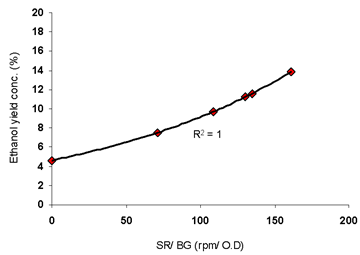• Figure 6. Coefficient of determination between ethanol yield and shaking rate- microbial growth ratio as predicted by derived model• Figure 7. Comparison of ethanol yields (relative to microbial growth) as obtained from experiment  and derived model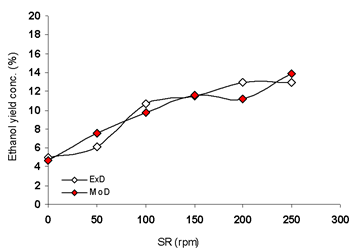• Figure 8. Comparison of area covered by ethanol yields (relative to shaking rate) as obtained from experiment  and derived model• Figure 9. Comparison of ethanol yields (relative to shaking rate- microbial growth ratio) as obtained from experiment  and derived model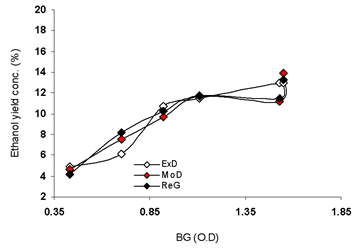• Figure 10. Comparison of ethanol yield (relative to microbial growth) as obtained from experiment  derived model and regression model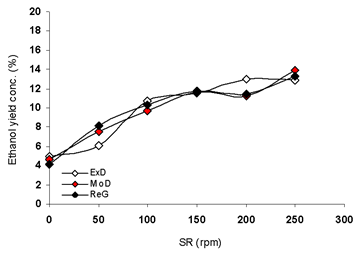• Figure 11. Comparison of areas covered by ethanol yield (relative to shaking rate) as obtained from experiment derived model and regression model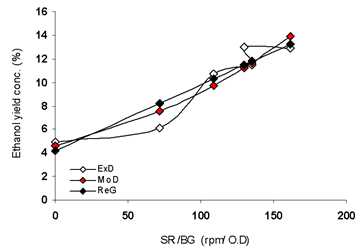• Figure 12. Comparison of ethanol yield (relative to shaking rate- microbial growth ratio) as obtained from experiment  derived model and regression model